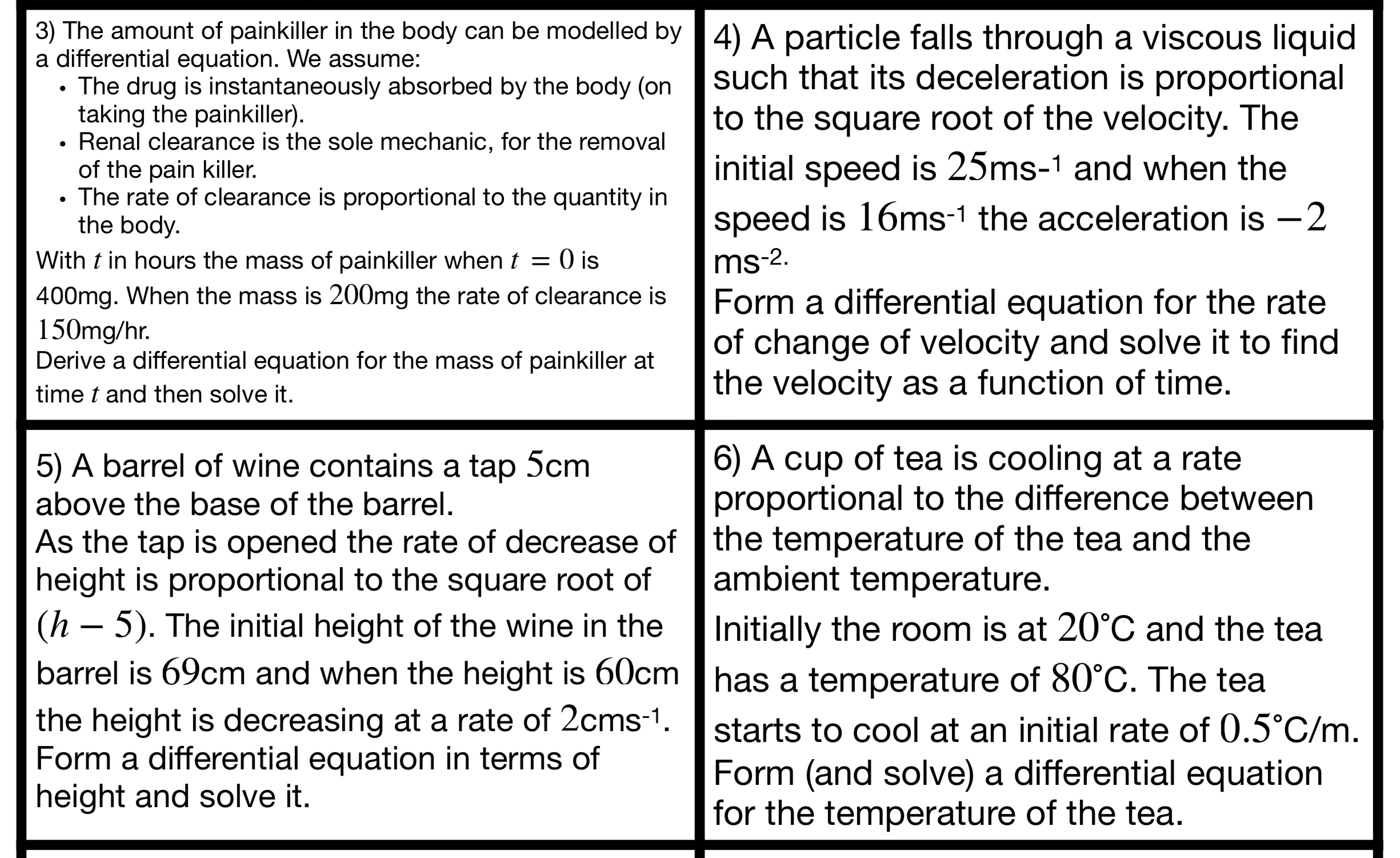Categories

# Modelling with Differential Equations

One thing my students (and I think most students) seem to struggle on is converting a given situation into a differential equation. The solving part seems to be relatively easy, assuming they have a good grip on integration, in comparison to this part!

As a revision activity I have made a card sort where students need to match a situation with a differential equation and then with the solution. To make it less obvious I have used $$x$$ for every dependent variable and $$y$$ for the corresponding independent variable.

A sample of some of the situations is shown below. They all lead to first order differential equations.Myself and Sheena (thank you for trialling it!) have used this so hopefully all the typos have now been found. The solutions are on page 3.